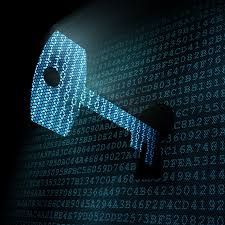# The Mysterious Code - II

Logic Level 2Locked inside a room, you see a Number Pad with this note:

"I am Professor Mad Math. The only way to escape is to punch in the correct Code into the Number Pad. The code is made up of the digits 1 to 9 inclusive, with each digit used exactly once.

• No two consecutive digits are adjacent to each other.

• The digits 2 and 4 are in the second and fourth position in some order.

• The digits 6 and 8 are in the sixth and eighth position in some order.

• The sum of the first five digits is 19.

• The sum of the last five digits is 27.

Can you crack the code?"

×